## How to calculate cross validation error using the Start and End Groups nodes in SAS

Started ‎10-31-2016 by
Modified ‎06-26-2017 by
Views 21,439

Cross validation is a widely-used model validation technique to estimate how accurately a predictive model will generalize to an independent data set. There are two main uses of cross validation: hyperparameter tuning and model assessment. This post will briefly discuss the use of cross validation in hyperparameter tuning before focusing on using cross validation for model assessment and showing how to compare models based on their cross validation errors using the Start Groups and End Groups nodes in SAS Enterprise Miner.

In hyperparameter tuning, cross validation is used to select the suitable flexibility of a model during model building. For example, when building a neural network model cross validation can be used to find optimal hyperparameter values (e.g., number of hidden layers, number of neurons, learning rate, momentum of stochastic gradient decent algorithm, etc.)  Hyperparameter tuning based on cross validation can be done automatically using the new Autotune statement available in a number of SAS® Visual Data Mining and Machine Learning procedures (PROCs FOREST, GRADBOOST, NNET, and TREESPLIT).

In model assessment, cross validation is used to compare different models that have already been built using the full training data. Suppose you built several models using various algorithms and hyperparameter settings and now you want to compare these models by estimating their prediction power. The basic idea in calculating cross validation error is to divide up training data into k-folds (e.g. k=5 or k=10).  Each fold will then be held out one at a time, the model will be trained on the remaining data, and that model will then be used to predict the target for the holdout observations.  When you finish fitting and scoring for all k versions of the training and validation data sets, you will obtain holdout predictions for all of the observations in your original training data. The average squared error between these predictions and the true observed response is the cross validation error.

In SAS Enterprise Miner, Start/End Groups nodes were originally implemented to stratify an analysis based on a stratification variable. However, with a couple of simple tricks these nodes can be used along with the Model Import node to obtain cross validation error of a model.  You can even compare several models based on their cross validation errors using the Model Comparison node.

Suppose you fit a model on your full training data using the Gradient Boosting node in SAS Enterprise Miner for the following set of the hyperparameters (Niterations=50, Shrinkage=0.2, Train proportion=60, etc):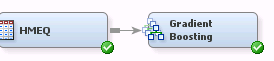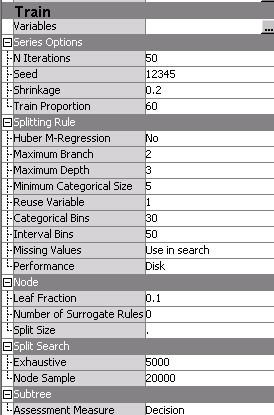Now you can calculate cross validation error of this model by running the following flow: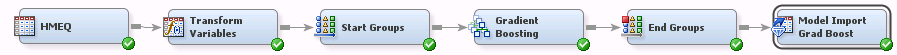1. Use Transform Variables node to create a k-fold cross validation indicator as a new input variable (_fold_) that randomly divides your data set into k-folds. Make sure to save this new variable as a segment variable. For example, for 5-fold cross validation, Formulas of the Transform Variables node should look like this: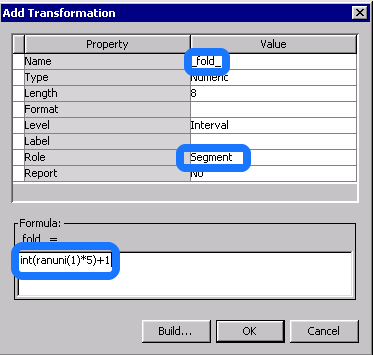2. In the Start Groups node, specify the “Mode” as “Cross-validation” and in the Gradient Boosting node make sure to use the same parameter settings that you used in your original boosted trees model. Run until the End Groups node.

While the Start/End Group nodes manage to create k versions of training data and calculate fit statistics of the training data, they do not actually calculate the cross validation error from scoring the holdout observations using these fitted models. However, if you check the score code generated by the End Groups node, you can see that it generates the correct score code to calculate the cross validation error. You can view this score code by first clicking the Results of the End Groups node, then on the top menu click View>>SAS Results>>Flow Code. However this readily available score code can be used by another node (such as Model Import node or SAS Code node) to obtain the cross validation error.

3. Attach the Model Import node and run the whole path. The Train: Average Squared Error column in the Results of the Model Import node is the k-fold cross validation error of your original boosted trees model that you trained by using the full training data.

If you are comparing multiple models based on their cross validation errors, your flow (attached as a zip file) should look like this: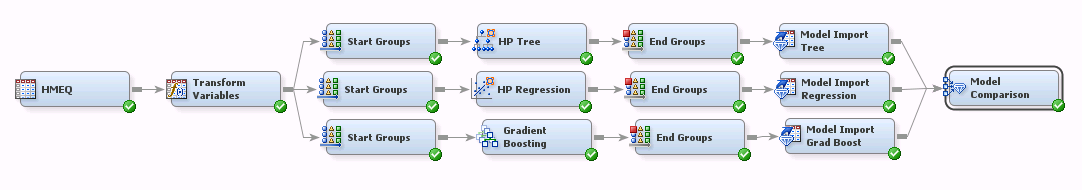Following table shows part of the output table that is produced by the Model Comparison: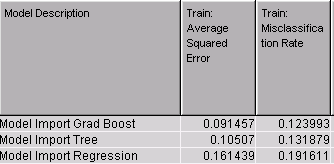Note that because the Model Import node is used, cross validation error is listed as Train: Average Squared Error.  But do not let the ‘Train:’ part confuse you --the Model Import uses the score code generated by the Start/End Groups node in the way we specified in (2), so it is actually the cross validation error. The output table above shows that cross validation error of the gradient boosting model is the smallest. If you choose this model to make prediction for a new data set, make sure to use the score code generated by your initial modeling node which builds the model on the full training set, instead of themodels that are built by the Start and End Groups nodes for the purpose of calculating the cross validation error.

I build on this diagram in another tip, Assessing Models by using k-fold Cross Validation in SAS Enterprise Miner, which shows how to obtain a 5-fold cross validation testing error, providing a more complete SAS Enterprise Miner flow.I had trouble to load your xml for this tip, but no issue for second tip you posted in May...Downloading xml is ok, but could not import to my EM to look. give me error message.

java.lang.NullPointerException
@ com.sas.analytics.eminer.impl.MiningNodeImpl.setMissingProperties(MiningNodeImpl.java:759)
@ com.sas.analytics.eminer.impl.MiningDGraphImpl.setMissingProperties(MiningDGraphImpl.java:190)
@ com.sas.analytics.eminer.impl.MiningWorkspaceImpl.setLayout(MiningWorkspaceImpl.java:1082)
@ com.sas.analytics.eminer.impl.MiningWorkspaceImpl.open(MiningWorkspaceImpl.java:1047)
@ com.sas.analytics.eminer.impl.MiningWorkspaceImpl.open(MiningWorkspaceImpl.java:980)
@ com.sas.analytics.eminer.visuals.MainDesktop.open(MainDesktop.java:727)
@ com.sas.analytics.eminer.visuals.MainDesktop.execute(MainDesktop.java:324)
@ com.sas.analytics.eminer.visuals.actions.WorkspaceAction.actionPerformed(WorkspaceAction.java:300)
@ com.sas.analytics.eminer.visuals.actions.EMAction.execute(EMAction.java:22)
@ com.sas.analytics.eminer.visuals.actions.ProjectAction.importWorkspaceInternal(ProjectAction.java:861)
@ com.sas.analytics.eminer.visuals.actions.ProjectAction.access\$300(ProjectAction.java:128)
@ com.sas.analytics.eminer.visuals.actions.ProjectAction\$5.run(ProjectAction.java:842)
@ com.sas.analytics.spkviewer.visuals.ProcessRunner\$1.run(ProcessRunner.java:36)
@ com.sas.analytics.spkviewer.visuals.util.SPKWaitWindow\$3.construct(SPKWaitWindow.java:242)
@ com.sas.analytics.spkviewer.visuals.util.SPKSwingWorker\$2.run(SPKSwingWorker.java:128)but for your tip2 xml, I don't have any trouble to import and view...Nice... Thanks for taking the time to write this up clearly.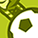Hey there,

Thanks for this useful explanation. I re-built the same structure for my data and it worked. Still, I have two questions regarding cross validation:

1. I would want to know how well my model(s) actually performed in terms of ROC (or misclassification rate as above) on average on the holdout sets. However, if I follow the structure above, the Model Comparison node only outputs the ROC (or misclassification) on train and not on the k test holdouts (correct?). How can I assess that?

2. What is the signification of cross validation error? What can I conclude from the fact that it is either small or large?

Any help would be highly appreciated!

Best regards,

DarioGreat questions, below are my answers:
1) Similar to "Train: Average Squared Error", "Train:AUC" is the AUC that is calculated by using the cross validation folds that are created from the training data.
2) Cross validation assessment statistics can be used in the same way you use validation or test data statistics, mainly to compare models.Dear @Funda_SAS ,

1. Could I say that the "Train:AUC" is the average AUC for the training data across all 10 folds? Since am actually wondering how my models perform on the validation set, I would want to get the average AUC that has been achieved on the 10 holdouts (rather than the average on the training folds). In case "Train:AUC" is indeed the average AUC of the 10 training folds, how would I have to modify the structure to get the average AUC of the 10 holdouts?

2. So, could I interpret a lower cross validation error as a smaller variance of the model's performance (e.g. accuracy or AUC), similar to the standard deviation or not?

Thanks your for quick clarifications 🙂Hi Funda,

many thanks for providing this detailed flow! I replicated it for the simple decision tree because the property "Cross Validation" of the Decision Tee node is a bit of a black box for me.

Below you find my results:

The _ASE_ of the Model Import node is equal to the _ASE_ of the End Group node where Group = _OVERALL_.

The _ASE_ of the _OVERALL_ Group however is calculated in accordance with the Flow Code which is for a 3-fold cross validation:

if ^(_fold_ =1)  then do; ...*prediction based on the tree trained on fold 2&3*

end;

if ^(_fold_ =2)  then do; ...*prediction based on the tree trained on fold 1&3*

end;

if ^(_fold_ =3)  then do; ...*prediction based on the tree trained on fold 1&2*

end;

But shouldn't be the code in the following way to obtain the precitions on the holdout samples?:

if (_fold_ =1)  then do; ...*prediction based on the tree trained on fold 2&3*

end;

if (_fold_ =2)  then do; ...*prediction based on the tree trained on fold 1&3*

end;

if (_fold_ =3)  then do; ...*prediction based on the tree trained on fold 1&2*

end;